COS 226 Seam Carving Programming Assignment checklist

Seam-carving is a content-aware image resizing technique where the image is reduced in size by one pixel of height (or width) at a time. A vertical seam in an image is a path of pixels connected from the top to the bottom with one pixel in each row; a horizontal seam is a path of pixels connected from the left to the right with one pixel in each column. Below left is the original 505-by-287 pixel image; below right is the result after removing 150 vertical seams, resulting in a 30% narrower image. Unlike standard content-agnostic resizing techniques (such as cropping and scaling), seam carving preserves the most interest features (aspect ratio, set of objects present, etc.) of the image.

Although the underlying algorithm is simple and elegant, it was not discovered until 2007. Now, it is now a core feature in Adobe Photoshop and other computer graphics applications.In this assignment, you will create a data type that resizes a W-by-H image using the seam-carving technique. Finding and removing a seam involves three parts and a tiny bit of notation:

1. Notation. In image processing, pixel (x, y) refers to the pixel in column x and row y, with pixel (0, 0) at the upper-left corner and pixel (W − 1, H − 1) at the lower-right corner. This is consistent with the Picture data type in algs4.jar.

 (0, 0) (1, 0) (2, 0) (0, 1) (1, 1) (2, 1) (0, 2) (1, 2) (2, 2) (0, 3) (1, 3) (2, 3)

Warning: this is the opposite of the standard mathematical notation used in linear algebra, where (i, j) refers to row i and column j and (0, 0) is at the lower-left corner.

We also assume that the color of each pixel is represented in RGB space, using three integers between 0 and 255. This is consistent with the java.awt.Color data type.

2. Energy calculation. The first step is to calculate the energy of a pixel, which is a measure of its importance—the higher the energy, the less likely that the pixel will be included as part of a seam (as you will see in the next step). In this assignment, you will use the dual-gradient energy function, which is described below. Here is the dual-gradient energy function of the surfing image above: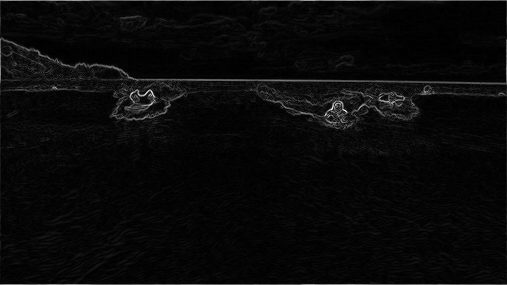The energy is high (white) for pixels in the image where there is a rapid color gradient (such as the boundary between the sea and sky and the boundary between the surfing Josh Hug on the left and the ocean behind him). The seam-carving technique avoids removing such high-energy pixels.

3. Seam identification. The next step is to find a vertical seam of minimum total energy. (Finding a horizontal seam is analogous.) This is similar to the classic shortest path problem in an edge-weighted digraph, but there are three important differences:

• The weights are on the vertices instead of the edges.

• The goal is to find the shortest path from any of the W pixels in the top row to any of the W pixels in the bottom row.

• The digraph is acyclic, where there is a downward edge from pixel (x, y) to pixels (x − 1, y + 1), (x, y + 1), and (x + 1, y + 1), assuming that the coordinates are in the prescribed ranges.

Seams cannot wrap around the image (e.g., a vertical seam cannot cross from the leftmost column of the image to the rightmost column).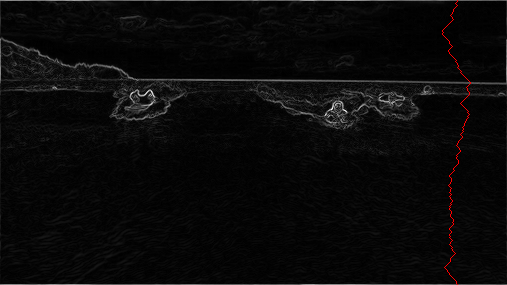4. Seam removal. The final step is remove from the image all of the pixels along the vertical or horizontal seam.

The SeamCarver API. Your task is to implement the following mutable data type:

public class SeamCarver {
public SeamCarver(Picture picture)                // create a seam carver object based on the given picture
public Picture picture()                          // current picture
public     int width()                            // width of current picture
public     int height()                           // height of current picture
public  double energy(int x, int y)               // energy of pixel at column x and row y
public   int[] findHorizontalSeam()               // sequence of indices for horizontal seam
public   int[] findVerticalSeam()                 // sequence of indices for vertical seam
public    void removeHorizontalSeam(int[] seam)   // remove horizontal seam from current picture
public    void removeVerticalSeam(int[] seam)     // remove vertical seam from current picture

public static void main(String[] args)            //  unit testing (required)
}


Corner cases. Your code must throw an exception when a constructor or method is called with an invalid argument, as documented below:

• By convention, the indices x and y are integers between 0 and W − 1 and between 0 and H − 1, respectively, where W is the width and H is the height of the current image. Throw a java.lang.IllegalArgumentException if energy() is called with either an x-coordinate or y-coordinate outside its prescribed range.

• Throw a java.lang.IllegalArgumentException if the constructor, removeVerticalSeam(), or removeHorizontalSeam() is called with a null argument.

• Throw a java.lang.IllegalArgumentException if either removeVerticalSeam() or removeHorizontalSeam() is called with an array of the wrong length or if the array is not a valid seam (either an entry is outside the height/width bounds or two adjacent entries differ by more than 1).

• Throw a java.lang.IllegalArgumentException if either removeVerticalSeam() or removeHorizontalSeam() is called when the width or height of the current picture is 1, respectively.

Constructor. The data type may not mutate the Picture argument to the constructor.

Computing the energy of a pixel. You will use the dual-gradient energy function: The energy of pixel $$(x, y)$$ is $$\sqrt{\Delta_x^2(x, y) + \Delta_y^2(x, y)}$$, where the square of the x-gradient $$\Delta_x^2(x, y) = R_x(x, y)^2 + G_x(x, y)^2 + B_x(x, y)^2$$, and where the central differences $$R_x(x, y)$$, $$G_x(x, y)$$, and $$B_x(x, y)$$ are the differences in the red, green, and blue components between pixel (x + 1, y) and pixel (x − 1, y), respectively. The square of the y-gradient $$\Delta_y^2(x, y)$$ is defined in an analogous manner. To handle pixels on the borders of the image, calculate energy by defining the leftmost and rightmost columns as adjacent and the topmost and bottommost rows as adjacent. For example, to compute the energy of a pixel (0, y) in the leftmost column, use its right neighbor (1, y) and its “left” neighbor (W − 1, y).

As an example, consider the 3-by-4 image (supplied as 3x4.png) with RGB values—each component is an integer between 0 and 255—as shown in the table below: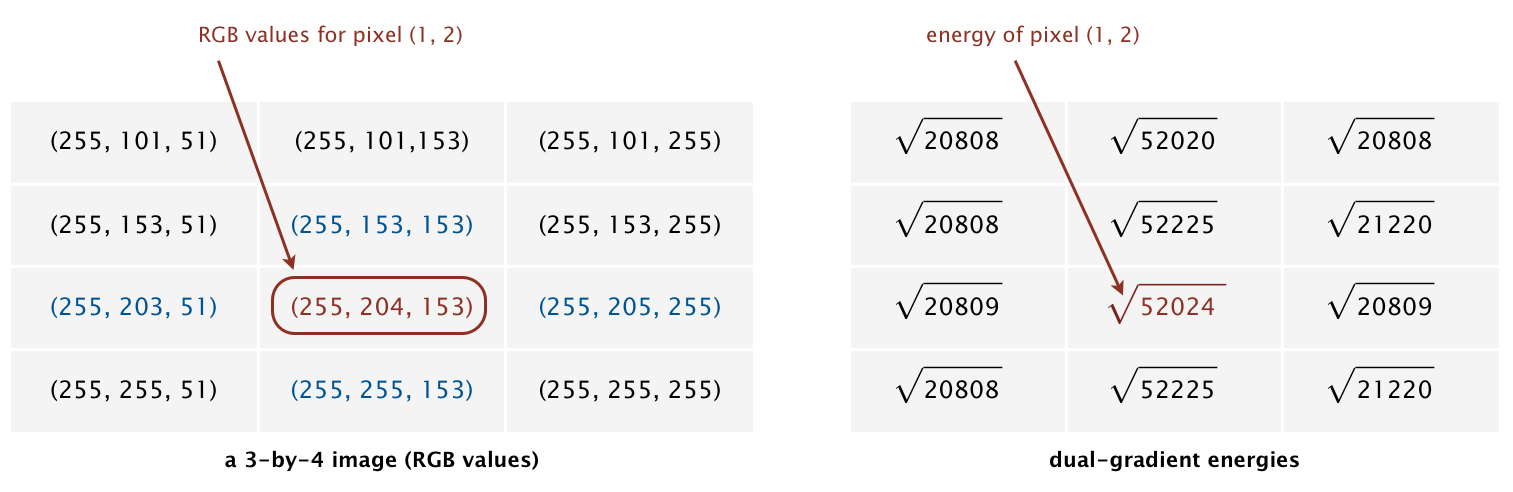• The energy of the non-border pixel (1, 2) is calculated from pixels (0, 2) and (2, 2) for the x-gradient

Rx(1, 2) = 255 − 255 = 0,
Gx(1, 2) = 205 − 203 = 2,
Bx(1, 2) = 255 − 51 = 204,
yielding Δx2(1, 2) = 22 + 2042 = 41620;

and pixels (1, 1) and (1, 3) for the y-gradient

Ry(1, 2) = 255 − 255 = 0,
Gy(1, 2) = 255 − 153 = 102,
By(1, 2) = 153 − 153 = 0,
yielding Δy2(1, 2) = 1022 = 10404.

Thus, the energy of pixel (1, 2) is $$\sqrt{41620 + 10404} = \sqrt{52024}$$. Similarly, the energy of pixel (1, 1) is $$\sqrt{204^2 + 103^2}= \sqrt{52225}$$.

• The energy of the border pixel (1, 0) is calculated by using pixels (0, 0) and (2, 0) for the x-gradient

Rx(1, 0) = 255 − 255 = 0,
Gx(1, 0) = 101 − 101 = 0,
Bx(1, 0) = 255 − 51 = 204,
yielding Δx2(1, 0) = 2042 = 41616;

and pixels (1, 3) and (1, 1) for the y-gradient

Ry(1, 0) = 255 − 255 = 0,
Gy(1, 0) = 255 − 153 = 102,
By(1, 0) = 153 − 153 = 0,
yielding Δy2(1, 0) = 1022 = 10404.

Thus, the energy of pixel (1, 0) is $$\sqrt{41616 + 10404} = \sqrt{52020}$$.

Finding a vertical seam. The findVerticalSeam() method returns an array of length H such that entry y is the column number of the pixel to be removed from row y of the image. For example, the dual-gradient energies of a 6-by-5 image (supplied as 6x5.png) are shown in the table below.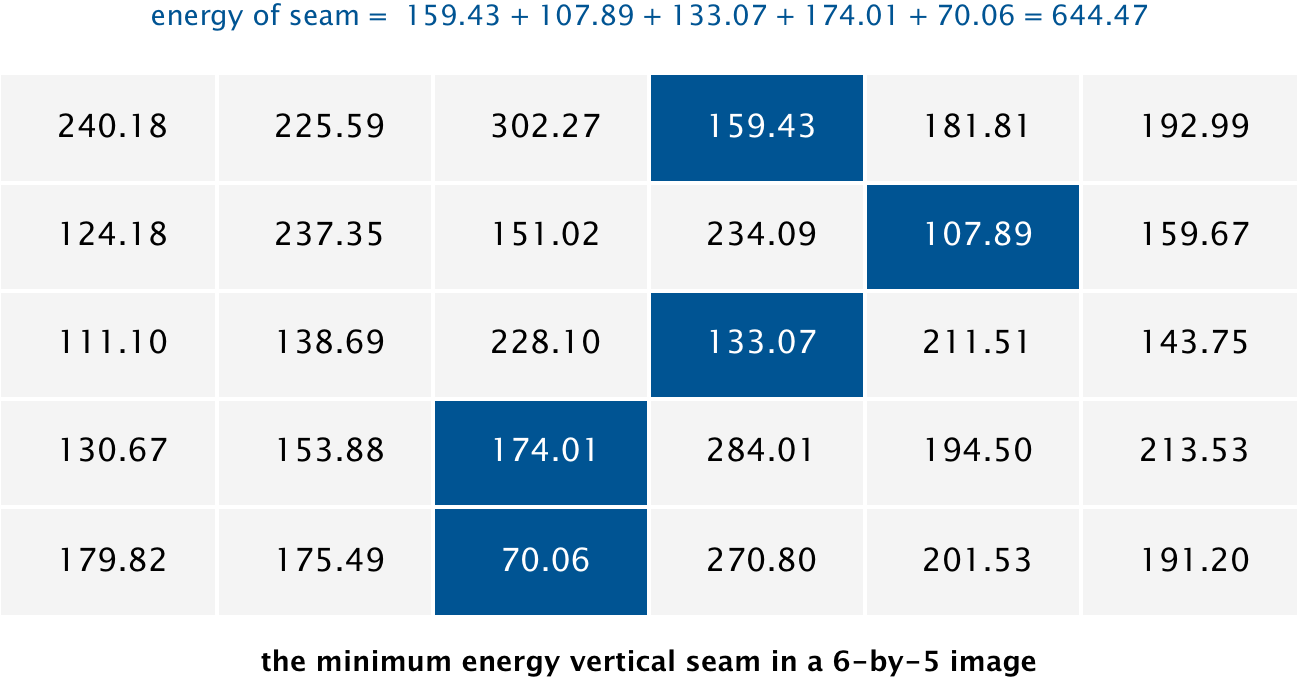The minimum energy vertical seam is highlighted in blue. In this case, the method findVerticalSeam() returns the array { 3, 4, 3, 2, 2 } because the pixels in the minimum energy vertical seam are (3, 0), (4, 1), (3, 2), (2, 3), and (2, 4). Remember that seams cannot wrap around the image. When there are multiple vertical seams with minimal total energy, your method can return any such seam.

Finding a horizontal seam. The behavior of findHorizontalSeam() is analogous to that of findVerticalSeam() except that it returns an array of length W such that entry x is the row number of the pixel to be removed from column x of the image. For the 6-by-5 image, the method findHorizontalSeam() returns the array { 2, 2, 1, 2, 1, 2 } because the pixels in the minimum energy horizontal seam are (0, 2), (1, 2), (2, 1), (3, 2), (4, 1), and (5, 2).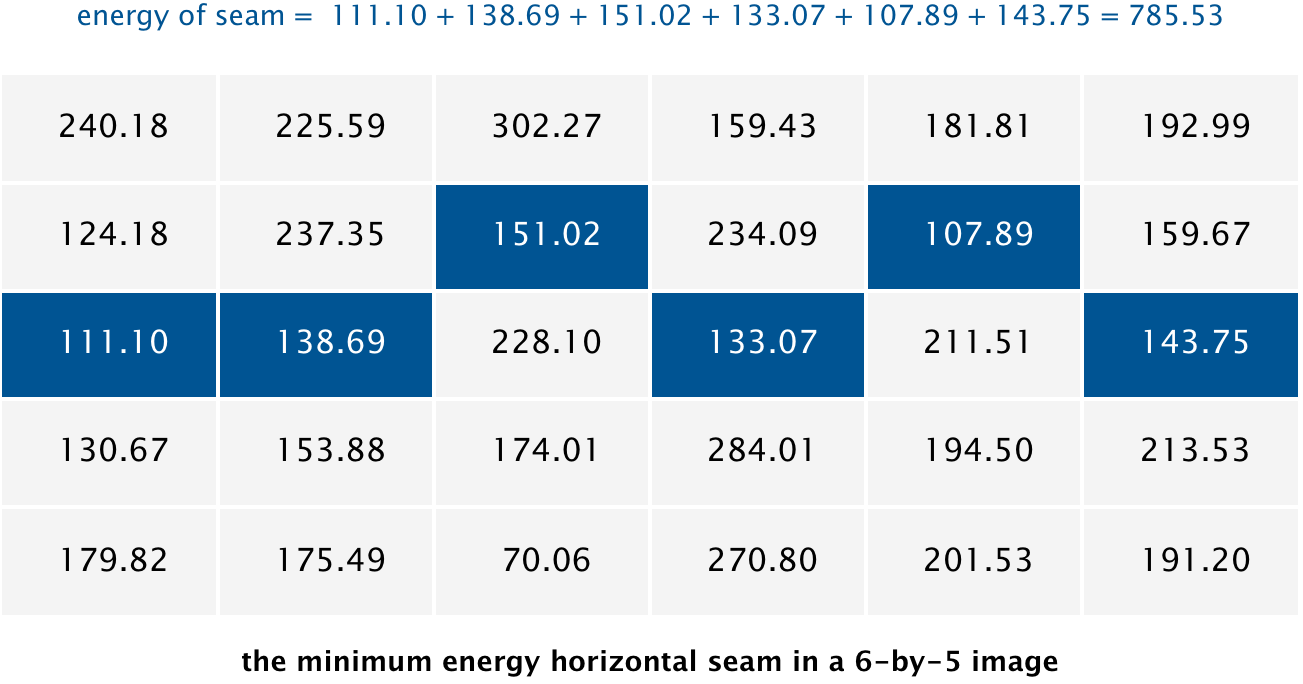Unit testing.  Your main() method must call each public constructor and method directly and help verify that they work as prescribed (e.g., by printing results to standard output).

Performance requirements. The width(), height(), and energy() methods must take constant time in the worst case. All other methods must run in time proportional to W H (or better) in the worst case.

Analysis of running time.  Estimate empirically the running times (in seconds) to remove one row and one column from a W-by-H image as a function of W, and H. Use tilde notation to simplify your answer.

Submission.  Submit SeamCarver.java, and any other files needed by your program (excluding those in algs4.jar). You may not call any library functions other than those in java.lang, java.util, java.awt.Color, and algs4.jar. Finally, submit a readme.txt file and answer the questions.

This assignment was developed by Josh Hug, Maia Ginsburg, and Kevin Wayne.